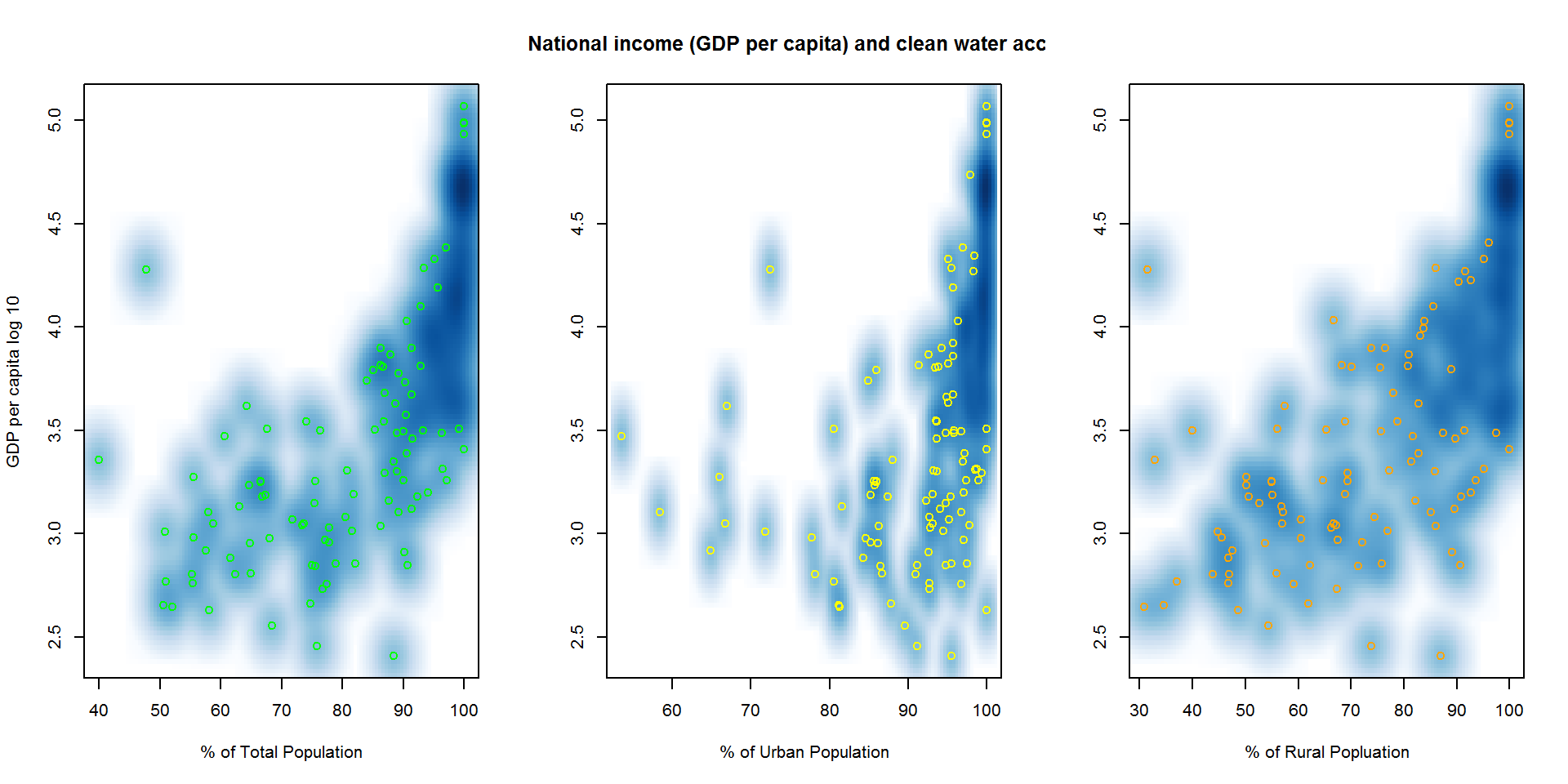This report explores the access to safe water supply around the world using the World Development Indicators of the World Bank. I use the report further in a blog post that discusses the Flint Water crisis.

## Data Processing

The analysis here starts from the premise that the data file obtained from the World Bank is present in the working directory of R. After reading the data, the column names are checked to facilitate further processing.

WDIdata <- read.csv("WDI Data/WDI_Data.csv")

### Creating the 2014 Water And Income Subset

The most recent year with data for both access to clean water and national income (GDP) is 2014. The data are shrunk to retain information for the two variables from 2014.

CountryName <- WDIdata[,"Country.Name"]
CountryCode <- WDIdata[,"Country.Code"]
IndicatorName <- WDIdata[,"Indicator.Name"]
IndicatorCode <- WDIdata[,"Indicator.Code"]
Year2014 <- WDIdata[, "X2014"]
WDI2014 <- data.frame(col1=CountryName, col2=CountryCode,
col3=IndicatorName, col4 = IndicatorCode, col5=Year2014)
Names2014 <- c("Country", "Country_Code", "Indicator","Indicator_Code", "2014_Values")
colnames(WDI2014) <- Names2014
H2Odata14 <- WDI2014[grep("*water*", WDI2014$Indicator), ] H2Odata <- H2Odata14[grep("^Improved*", H2Odata14$Indicator), ]
GDPdata14 <- WDI2014[grep("^GDP*", WDI2014$Indicator), ] GDPdata <- GDPdata14[grep("*current*", GDPdata14$Indicator),]
GDPdata <- GDPdata[grep("*capita*", GDPdata$Indicator),] GDPdata <- GDPdata[grep("US", GDPdata$Indicator),]

### Melting with “reshape”

The data now need to be reformatted in a way that each indicator is a variable (column) and each country is a case (row). There are three safe water variables (rural, urban, and total) and one national income variable (GDP per capita). The tidy data includes all four variables.

library(reshape2)
H2Omelt14 <- dcast(H2Odata, Country ~ Indicator_Code, value.var = '2014_Values')
NamesH2O <- c("Country_or_Area", "H2O_Rural_Percent", "H2O_Urban_Percent", "H2O_Total_Percent")
colnames(H2Omelt14) <- NamesH2O
GDPmelt14 <- dcast(GDPdata, Country ~ Indicator_Code, value.var = '2014_Values')
NamesGDP <- c("Country_or_Area", "GDP_per_capita_in_current_USD")
colnames(GDPmelt14) <- NamesGDP
#Removing NAs
Data14NAS <- merge(H2Omelt14, GDPmelt14, by = "Country_or_Area")
Data14 <- subset(Data14NAS, !is.na(Data14NAS\$GDP_per_capita_in_current_USD))
shortColNames <- c("Country", "H2O_Rur", "H2O_Urb", "H2O_Tot", "GDP_Cap")
colnames(Data14) <- shortColNames

### Creating Total, Urban And Rural Data Subsets

Next, to facilitate plot construction and comparison, the tidy data are subset into rural, urban and total.

Data14total <- Data14[, c(1, 4, 5)]
rownames(Data14total) <- Data14total[,1]
Data14total <- Data14total[,-1]
Data14total <- na.omit(Data14total)

Data14rural <- Data14[, c(1, 2, 5)]
rownames(Data14rural) <- Data14rural[,1]
Data14rural <- na.omit(Data14rural)
Data14rural <- Data14rural[,-1]

Data14urban <- Data14[, c(1, 3, 5)]
rownames(Data14urban) <- Data14urban[,1]
Data14urban <- na.omit(Data14urban)
Data14urban <- Data14urban[,-1]

# Results

The results suggest that there is a general tendency for countries with higher per capita income to provide access to clean water to overwhelming majorities of their populations. Further, some countries with moderate or low GDP per capita are also able to secure access to clean water to high percentages of their populations. Within some countries, there seems to be a difference between access to clean water in rural (relatively worse) and urban areas (relatively better). The plot comparison suggests that the patterns in the urban data drive the patterns in the total data.

par(mfrow=c(1,3))
with(Data14total, smoothScatter(H2O_Tot, log10(GDP_Cap),
colramp = colorRampPalette(c("white", blues9)),
xlab = "% of Total Population",
ylab = "GDP per capita log 10",
col = "green", pch = 21))
with(Data14urban, smoothScatter(H2O_Urb, log10(GDP_Cap),
colramp = colorRampPalette(c("white", blues9)),
xlab = "% of Urban Population",
ylab = "",
main = "National income (GDP per capita) and clean water access",
col = "yellow", pch = 21))
with(Data14rural, smoothScatter(H2O_Rur, log10(GDP_Cap),
colramp = colorRampPalette(c("white", blues9)),
xlab = "% of Rural Popluation",
ylab = "",
col = "orange", pch = 21))